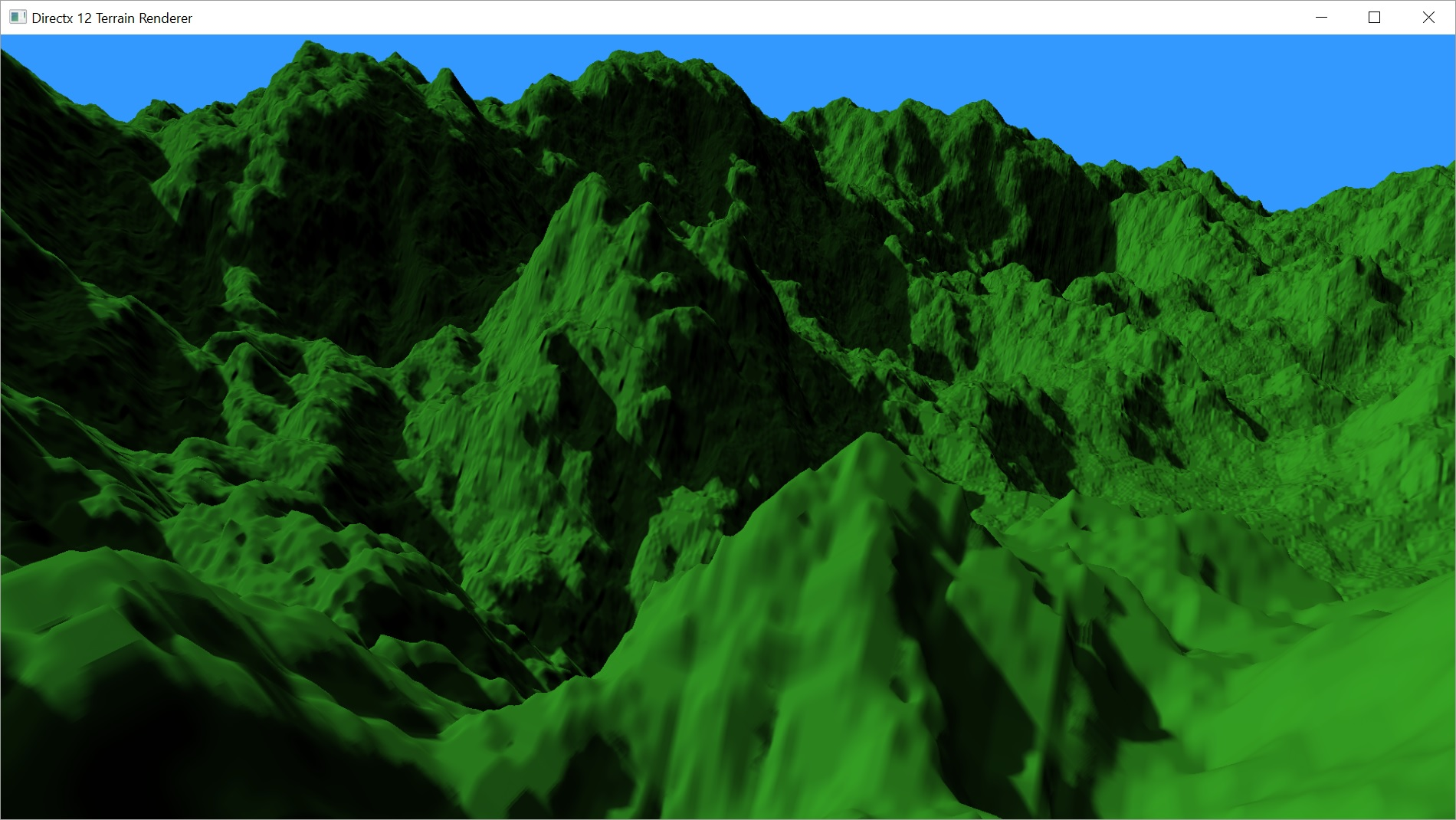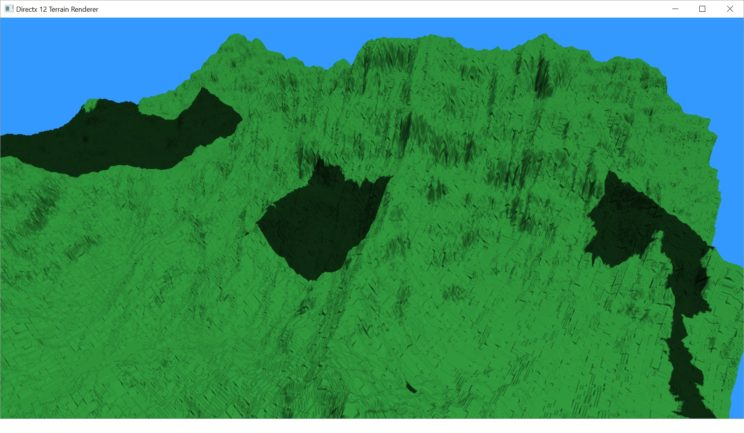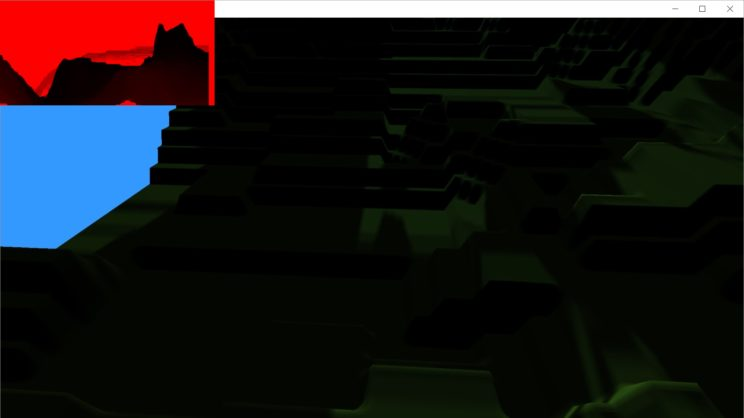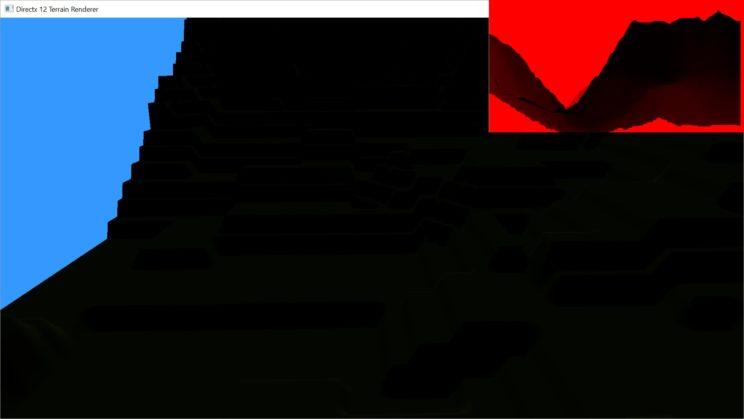# Rendering Terrain Part 17 – More CSM and Fixing Mistakes

Last post, I got started on implementing Cascaded Shadow Maps. I got as far as turning the original shadow map into a shadow atlas and defining the new matrices that transform points into light space.
The problem is that the solution I came up with to get the view frustum used to calculate those matrices was wrong. It was giving me bogus results that became obvious when I tried to actually apply the shadow cascades to the terrain.

### Mistakes

So before we move on to talk about getting the shadows actually displaying, let’s fix my mistakes. I actually made a couple of mistakes. I don’t have screenshots of each mistake, just one with all of them in.If we look back at the first chunk of code for calculating the view frustum, we can see two of my mistakes in the first few lines of code:

```void Camera::GetBoundingSphereByNearFar(float near, float far, XMFLOAT4& center, float& radius) {
// get the current view matrix
// calculate the projection matrix based on the supplied near/far planes.
XMMATRIX proj = XMMatrixPerspectiveFovLH(XMConvertToRadians(60.0f), (float)mWidth / (float)mHeight, near, far);
XMMATRIX viewproj = view * proj;
XMMATRIX invViewProj = XMMatrixInverse(nullptr, viewproj); // the inverse view/projection matrix

// 3 points on the unit cube representing the view frustum in view space.
XMVECTOR nlb = XMLoadFloat4(&XMFLOAT4(-1.0f, -1.0f, -1.0f, 1.0f));
XMVECTOR flb = XMLoadFloat4(&XMFLOAT4(-1.0f,  1.0f,  1.0f, 1.0f));
XMVECTOR frt = XMLoadFloat4(&XMFLOAT4( 1.0f,  1.0f,  1.0f, 1.0f));

// transform the frustum into world space.
nlb = XMVector3Transform(nlb, invViewProj);
flb = XMVector3Transform(flb, invViewProj);
frt = XMVector3Transform(frt, invViewProj);
...
}```

Well, actually there may be only one mistake here, but the way I’m doing things now is so different, I assumed I had made another. I’ll go over each of them.
My first mistake was in thinking that I could use XMMatrixPerspectiveFovLH() with different near and far planes and get frustums that would line up with each other. When I took a look at the values for one cascade’s far plane, they should have matched up exactly with the near values for the next cascade. They wound up being vastly different. Because I made a few other mistakes at the same time, I’m not actually entirely clear what problems exactly this caused, but I can guess that not having the cascades lined up would result in a lot of missing shadows, or extra shadows where there should not have been any.
The second mistake may not be a mistake, after all. In this code, I multiply the view and projection matrices together and then take the inverse. I apply the inverse to the frustum as it would appear in view/projection space, which should be a unit cube.
I found this really helpful tutorial which has a different way of defining the frustum, only defining it in view space, rather than in view/projection space. When I was working on implementing this method, I thought my method was wrong, but I don’t think it necessarily is. With the correct projection matrix calculation, you could probably make this work. But we only really need the field of view to calculate the frustum points, so why bother with the whole projection matrix?
I also created a Frustum struct to hold all of the frustum and bounding sphere data, just to make it easier to pass around in case I need the frustum itself later.

```struct Frustum {
XMFLOAT3 nlb;
XMFLOAT3 nlt;
XMFLOAT3 nrb;
XMFLOAT3 nrt;
XMFLOAT3 flb;
XMFLOAT3 flt;
XMFLOAT3 frb;
XMFLOAT3 frt;
XMFLOAT3 center;
};

Frustum Camera::CalculateFrustumByNearFar(float near, float far) {
Frustum f;

float tanHalfHFOV = tanf(XMConvertToRadians(mHFOV / 2.0f));
float tanHalfVFOV = tanf(XMConvertToRadians(mVFOV / 2.0f));

float xNear = near * tanHalfHFOV;
float xFar = far * tanHalfHFOV;
float yNear = near * tanHalfVFOV;
float yFar = far * tanHalfVFOV;

f.nlb = XMFLOAT3(-xNear, -yNear, near);
f.nrb = XMFLOAT3( xNear, -yNear, near);
f.nlt = XMFLOAT3(-xNear,  yNear, near);
f.nrt = XMFLOAT3( xNear,  yNear, near);

f.flb = XMFLOAT3(-xFar, -yFar, far);
f.frb = XMFLOAT3( xFar, -yFar, far);
f.flt = XMFLOAT3(-xFar,  yFar, far);
f.frt = XMFLOAT3( xFar,  yFar, far);

// get the current view and projection matrices
XMMATRIX viewproj = view;
XMMATRIX invViewProj = XMMatrixInverse(nullptr, viewproj); // the inverse view/projection matrix

nlb = XMVector3Transform(nlb, invViewProj);
nrb = XMVector3Transform(nrb, invViewProj);
nlt = XMVector3Transform(nlt, invViewProj);
nrt = XMVector3Transform(nrt, invViewProj);
flb = XMVector3Transform(flb, invViewProj);
frb = XMVector3Transform(frb, invViewProj);
flt = XMVector3Transform(flt, invViewProj);
frt = XMVector3Transform(frt, invViewProj);

XMFLOAT4 _nlb, _nrb, _nrt, _nlt, _flb, _frt, _frb, _flt;
XMStoreFloat4(&_nlb, nlb);
XMStoreFloat4(&_nrb, nrb);
XMStoreFloat4(&_nlt, nlt);
XMStoreFloat4(&_nrt, nrt);
XMStoreFloat4(&_flb, flb);
XMStoreFloat4(&_frb, frb);
XMStoreFloat4(&_flt, flt);
XMStoreFloat4(&_frt, frt);

nlb = nlb / _nlb.w;
nrb = nrb / _nrb.w;
nlt = nlt / _nlt.w;
nrt = nrt / _nrt.w;
flb = flb / _flb.w;
frb = frb / _frb.w;
flt = flt / _flt.w;
frt = frt / _frt.w;

XMStoreFloat3(&f.nlb, nlb);
XMStoreFloat3(&f.nrb, nrb);
XMStoreFloat3(&f.nlt, nlt);
XMStoreFloat3(&f.nrt, nrt);
XMStoreFloat3(&f.flb, flb);
XMStoreFloat3(&f.frb, frb);
XMStoreFloat3(&f.flt, flt);
XMStoreFloat3(&f.frt, frt);

return f;
}

void FindBoundingSphere(XMFLOAT3 a, XMFLOAT3 b, XMFLOAT3 c, XMFLOAT3& center, float& radius) {
XMVECTOR ac = _c - _a;
XMVECTOR ab = _b - _a;
XMVECTOR N = XMVector3Normalize(XMVector3Cross(ab, ac));
XMVECTOR halfAB = _a + ab * 0.5f;
XMVECTOR halfAC = _a + ac * 0.5f;
XMVECTOR perpAB = XMVector3Normalize(XMVector3Cross(ab, N));
XMVECTOR perpAC = XMVector3Normalize(XMVector3Cross(ac, N));
// line,line intersection test. Line 1 origin: halfAB, direction: perpAB; Line 2 origin: halfAC, direction: perpAC
N = XMVector3Cross(perpAB, perpAC);
XMVECTOR SR = halfAB - halfAC;
XMFLOAT4 _N, _SR, _E;
XMStoreFloat4(&_N, N);
XMStoreFloat4(&_SR, SR);
XMStoreFloat4(&_E, perpAC);
float absX = fabs(_N.x);
float absY = fabs(_N.y);
float absZ = fabs(_N.z);
float t;
if (absZ > absX && absZ > absY) {
t = (_SR.x * _E.y - _SR.y * _E.x) / _N.z;
}
else if (absX > absY) {
t = (_SR.y * _E.z - _SR.z * _E.y) / _N.x;
}
else {
t = (_SR.z * _E.x - _SR.x * _E.z) / _N.y;
}

XMVECTOR Circumcenter = halfAB - t * perpAB;
XMVECTOR r = XMVector3Length(_c - Circumcenter);

XMStoreFloat3(&center, Circumcenter);
}```

The bit at the beginning is the important bit. Most of the rest is just moving data between the variable types necessary to manipulate it. I also broke the bounding sphere calculation into a separate function. It will probably end up in some sort of bounding volume library eventually.

### Getting CSM Working-ish

As of last post, we were capable of drawing the same thing to each map and could, albeit brokenly, define a single full size cascade. Now we need to actually create the four cascades.
The first thing I did was change the shadow pass to use a different constant buffer from the normal pass. In our current version of the shadow pass, we just need the View/Projection matrix to transform the vertices and the camera’s eye to calculate the dynamic level of detail.

```struct ShadowMapShaderConstants {
XMFLOAT4	eye;
};```

There’s no point sending the rest of the data in and we’d need to have a lot of extra unnecessary fields as you can’t change the values within the buffer while it is in use.
For the same reason, I also actually created four of these little shadow constant buffers, one for each cascade. We just set the values in the correct one on each pass and then bind it.

```void Scene::DrawShadowMap(ID3D12GraphicsCommandList* cmdList) {

cmdList->ClearDepthStencilView(mlDescriptorHeaps->GetCPUDescriptorHandleForHeapStart(), D3D12_CLEAR_FLAG_DEPTH, 1.0f, 0, 0, nullptr);
cmdList->OMSetRenderTargets(0, nullptr, false, &mlDescriptorHeaps->GetCPUDescriptorHandleForHeapStart());

cmdList->SetPipelineState(mlPSOs);
cmdList->SetGraphicsRootSignature(mlRootSigs);

ID3D12DescriptorHeap* heaps[] = { mlDescriptorHeaps };
cmdList->SetDescriptorHeaps(_countof(heaps), heaps);

// set the srv buffer.
CD3DX12_GPU_DESCRIPTOR_HANDLE handleSRV(mlDescriptorHeaps->GetGPUDescriptorHandleForHeapStart(), 2, msizeofCBVSRVDescHeapIncrement);
cmdList->SetGraphicsRootDescriptorTable(0, handleSRV);

// set the terrain shader constants
CD3DX12_GPU_DESCRIPTOR_HANDLE handleCBV(mlDescriptorHeaps->GetGPUDescriptorHandleForHeapStart(), 1, msizeofCBVSRVDescHeapIncrement);
cmdList->SetGraphicsRootDescriptorTable(1, handleCBV);

for (int i = 0; i < 4; ++i) {

constants.eye = C.GetEyePosition();
// there are now a total of 8 resources in the heap. Indices 4-7 are the shadow map constants.
CD3DX12_GPU_DESCRIPTOR_HANDLE handleShadowCBV(mlDescriptorHeaps->GetGPUDescriptorHandleForHeapStart(), 4 + i, msizeofCBVSRVDescHeapIncrement);

// mDrawMode = 0/false for 2D rendering and 1/true for 3D rendering
T.Draw(cmdList, true);
}

}```

This leads to a bit simpler PerFrameConstantBuffer. We still need the camera’s position and view/projection matrix, as well as the view frustum for culling. Now we just need the four matrices to transform into each of the cascades’ texture space.

```struct PerFrameConstantBuffer {
XMFLOAT4X4	viewproj;
XMFLOAT4	eye;
XMFLOAT4	frustum;
LightSource light;
};```

Speaking of the texture space, on my first pass through, I got some weird results, which are part of what was causing the above image to look so funky. This wasn’t a mistake I had made in the previous post, but just something that was correct for a single shadow map, but wrong for multiple. Our original texture space matrix T looked like this:

```	// transform NDC space [-1, +1]^2 to texture space [0, 1]^2
XMMATRIX T(0.5f,  0.0f, 0.0f, 0.0f,
0.0f, -0.5f, 0.0f, 0.0f,
0.0f,  0.0f, 1.0f, 0.0f,
0.5f,  0.5f, 0.0f, 1.0f);
```

That gets multiplied with the light’s view/projection matrix to get to texture space for a single shadow map taking up the entire chunk of memory. Now, we want it to only take up one quarter of the same memory.

```XMMATRIX T(0.25f, 0.0f, 0.0f, 0.0f,
0.0f, -0.25f, 0.0f, 0.0f,
0.0f, 0.0f, 1.0f, 0.0f,
x, y, 0.0f, 1.0f);```

x and y are defined based on which cascade we’re looking at.
We’ll get to that, but first, let’s assume for a second that we’re done with the DirectX code and look at the shader code.

Our Vertex and Hull shaders don’t need to change at all. There are some changes to the Domain and Pixel shaders.
For the Domain shader, we slightly change our DS_OUTPUT structure. We need to multiply our vertex positions by each of the four shadow matrices and pass them all to the Pixel shader. We need to pass all four because we can only get correct interpolation between vertices this way. If you try to do the multiplications in the Pixel shader, the article ‘Practical Cascaded Shadow Maps’ by Zhang, Zapriagaev, and Bentham from ShaderX7 proves your results will be mathematically incorrect.
On the other hand, you can’t select the the cascade in the Domain shader because the triangle you are looking at may cross cascades.
So the new/changed bits look like the below. I left out most of the shader for brevity.

```struct DS_OUTPUT
{
float4 pos : SV_POSITION;
float3 worldpos : POSITION;
float2 tex : TEXCOORD4;
};

DS_OUTPUT main(
HS_CONSTANT_DATA_OUTPUT input,
float2 domain : SV_DomainLocation,
const OutputPatch<HS_CONTROL_POINT_OUTPUT, NUM_CONTROL_POINTS> patch)
{
DS_OUTPUT output;

output.worldpos = lerp(lerp(patch.worldpos, patch.worldpos, domain.x), lerp(patch.worldpos, patch.worldpos, domain.x), domain.y);

...

[unroll]
for (int i = 0; i < 4; ++i) {
// generate projective tex-coords to project shadow map onto scene.

}
return output;
}```

In the Pixel shader, we use the exact same calcShadowFactor function that we defined back in Part 13. We just need to add a function to pick which cascade to use.

```float4 decideOnCascade(float4 shadowpos) {
// if shadowpos.xy is in the range [0, 0.5], then this point is in the first cascade
}

}

}

}

float4 main(DS_OUTPUT input) : SV_TARGET
{
float3 norm = estimateNormal(input.tex);

float4 color = float4(0.22f, 0.72f, 0.31f, 1.0f);

float4 diffuse = max(shadowfactor, light.amb) * light.dif * dot(-light.dir, norm);
float3 V = reflect(light.dir, norm);
float3 toEye = normalize(eye.xyz - input.worldpos);
float4 specular = shadowfactor * 0.1f * light.spec * pow(max(dot(V, toEye), 0.0f), 2.0f);

return (diffuse + specular) * color;
}```

I can’t imagine a simpler way to pick the cascade. Just find the cascade where the shadow position calculated with the corresponding matrix resulted in a value that falls in the correct quarter of the atlas. If it doesn’t fall in that quarter, then that isn’t the correct cascade. We use the fourth cascade as a fall back.
With these new additions to the shaders, we are almost getting the results we want. There’s just one thing we’ve missed.If we move the eye slightly, we can see what the above picture should have looked like.The images are pretty dark. Sorry about that. But you can probably tell that the first one has gaps and the second is solid shadow. You can also see the differences in the shadow maps produced in the red corners. There appears to be a pretty big piece missing from the first image.
The cause of this is that the terrain is being clipped to our new camera frustum. Remember that our original camera view frustum was based on the radius of the bounding sphere of the entire scene. Now, each cascade’s light view frustum is based on the radius of that cascade’s camera view frustum, which is much smaller. Any shadow casters outside of that radius wind up not casting shadows.
Here’s the near and far values I’m using for the first three cascades. The array reads near, near/far, near/far, far. I left out values for the fourth cascade because I decided that cascade would default back to the original full scene shadow map, to ensure we have shadow everywhere all the time.

`static const float CASCADE_PLANES[] = { 0.1f, 64.0f, 128.0f, 256.0f };`

The fix for this was pretty simple. When we define the light view frustum, we define it like so:

```float l = spherecenterls.x - radius;
float b = spherecenterls.y - radius;
float n = spherecenterls.z - radius;
float r = spherecenterls.x + radius;
float t = spherecenterls.y + radius;
float f = spherecenterls.z + radius;
XMMATRIX P = XMMatrixOrthographicOffCenterLH(l, r, b, t, n, f);```

This is a cube centered on the center of our cascade, with the radius set to the radius of the bounding sphere of the cascade’s frustum. If we change the near and far planes (n, f) to use the radius of the bounding sphere of the whole scene, we’ll eliminate the clipping. The other values seem to be correct and the results I get are decent.
Here’s the CalculateShadowMatrices() method as it currently stands:

```void DayNightCycle::CalculateShadowMatrices(XMFLOAT3 centerBS, float radiusBS, Camera* cam) {
LightSource light = mdlSun.GetLight();

XMVECTOR lightpos = targetpos - 2.0f * radiusScene * lightdir;

XMVECTOR up = XMVectorSet(0.0f, 1.0f, 0.0f, 0.0f);
up = XMVector3Cross(up, lightdir);

XMMATRIX V = XMMatrixLookAtLH(lightpos, targetpos, up); // light space view matrix transform bounding sphere to light space
XMFLOAT4 spherecenterls;

// create the first three cascades.
for (int i = 0; i < 3; ++i) {

XMStoreFloat4(&spherecenterls, XMVector3TransformCoord(c, V));

// orthographic frustum
float l = spherecenterls.x - radius;
float b = spherecenterls.y - radius;
float n = spherecenterls.z - radiusScene;
float r = spherecenterls.x + radius;
float t = spherecenterls.y + radius;
float f = spherecenterls.z + radiusScene;
XMMATRIX P = XMMatrixOrthographicOffCenterLH(l, r, b, t, n, f);

XMMATRIX S = V * P;

XMFLOAT2 so;
XMVECTOR rounding = roundedOrigin - shadowOrigin;
XMStoreFloat2(&so, rounding);
XMMATRIX roundMatrix = XMMatrixTranslation(so.x, so.y, 0.0f);
S *= roundMatrix;

// transform NDC space [-1, +1]^2 to texture space [0, 1]^2
float x, y;
if (i == 0) {
x = 0.25f;
y = 0.25f;
} else if (i == 1) {
x = 0.25f;
y = 0.75f;
} else {
x = 0.75f;
y = 0.25f;
}

XMMATRIX T(0.25f, 0.0f, 0.0f, 0.0f,
0.0f, -0.25f, 0.0f, 0.0f,
0.0f, 0.0f, 1.0f, 0.0f,
x, y, 0.0f, 1.0f);

S *= T;

}

// create the fourth cascade as just a full scene shadow map.
XMStoreFloat4(&spherecenterls, XMVector3TransformCoord(c, V));

// orthographic frustum
float l = spherecenterls.x - radiusScene;
float b = spherecenterls.y - radiusScene;
float n = spherecenterls.z - radiusScene;
float r = spherecenterls.x + radiusScene;
float t = spherecenterls.y + radiusScene;
float f = spherecenterls.z + radiusScene;
XMMATRIX P = XMMatrixOrthographicOffCenterLH(l, r, b, t, n, f);

XMMATRIX S = V * P;

XMFLOAT2 so;

XMVECTOR rounding = roundedOrigin - shadowOrigin;
XMStoreFloat2(&so, rounding);
XMMATRIX roundMatrix = XMMatrixTranslation(so.x, so.y, 0.0f);
S *= roundMatrix;

// transform NDC space [-1, +1]^2 to texture space [0, 1]^2
XMMATRIX T(0.25f,  0.0f, 0.0f, 0.0f,
0.0f, -0.25f, 0.0f, 0.0f,
0.0f,  0.0f, 1.0f, 0.0f,
0.75f,  0.75f, 0.0f, 1.0f);
S *= T;